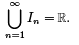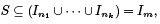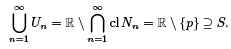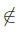Heine Borel - Sequences and Series, CSIR-NET Mathematical Sciences

# Heine Borel - Sequences and Series, CSIR-NET Mathematical Sciences - Notes | Study Mathematics for IIT JAM, CSIR NET, UGC NET - Mathematics

 1 Crore+ students have signed up on EduRev. Have you?

Theorem (Heine-Borel Theorem): A subset S of R is compact if and only if S is closed and bounded. Proof. First we suppose that S is compact. To see that S is bounded is fairly simple: Let In = (−n, n). ThenTherefore S is covered by the collection of {In }. Hence, since S is compact, ﬁnitely many will suﬃce.where m = max{n1, . . . , nk}. Therefore |x| ≤ m for all x ∈ S , and S is bounded.

Now we will show that S is closed. Suppose not. Then there is some point p ∈ (cl S) \ S . For each n, deﬁne the neighborhood around p of radius 1/n, Nn = N (p, 1/n). Take the complement of the closure of Nn, Un  = R \ cl Nn . Then Un is open (since its complement is closed), and we haveTherefore, {Un} is an open cover for S . Since S is compact, there is a ﬁnite subcover {Un1 , � � � , Unk} for S . Furthermore, by the way they are constructed, Ui ⊆ Uj if i ≤ j . It follows that S ⊆ Um where m = max{n1, . . . , nk}. But then S ∩ N (p, 1/m)  = �, which contradicts our choice of p ∈ (cl S) \ S .

Conversely, we want to show that if S is closed and bounded, then S is compact. Let F be an open cover for S . For each x ∈ R, deﬁne the set

Sx = S ∩ (−∞, x],

and let

B = {x : Sx is covered by a ﬁnite subcover of F}.

Since S is closed and bounded, our lemma tells us that S has both a maximum and a minimum. Let d = min S . Then Sd = {d} and this is certainly covered by a ﬁnite subcover of F. Therefore, d ∈ B and B is nonempty. If we can show that B is not bounded above, then it will contain a number p greater than max S .
But then, Sp = S so we can conclude that S is covered by a ﬁnite subcover, and is therefore compact.

Toward this end, suppose that B is bounded above and let m = sup B. We shall show that m ∈ S and mS both lead to contradictions.

If m ∈ S , then since F is is an open cover of S , there exists F0 in F such that m ∈ F0 . Since F0 is open there exists an interval [x1 , x2] in F0 such that

x1 < m < x2.

Since x1 < m and m = supB , there exists F1, . . . , Fk in F that cover Sx1 . But then F0 , F1 , . . . , Fk cover Sx2 , so that x2 ∈ B. But this contradicts m = sup B.

If m ∉ S , then since S is closed there exists ε > 0 such that N (m, ε) ∩ S = �. But then

Sm−ε = Sm+ε.

Since m − ε ∈ B we have m + ε ∈ B, which again contradicts m = sup B.

Therefore, either way, if B is bounded above, we get a contradiction. We conclude that B is not bounded above, and S must be compact.

The document Heine Borel - Sequences and Series, CSIR-NET Mathematical Sciences - Notes | Study Mathematics for IIT JAM, CSIR NET, UGC NET - Mathematics is a part of the Mathematics Course Mathematics for IIT JAM, CSIR NET, UGC NET.
All you need of Mathematics at this link: Mathematics

## Mathematics for IIT JAM, CSIR NET, UGC NET

559 videos|198 docs
 Use Code STAYHOME200 and get INR 200 additional OFF

## Mathematics for IIT JAM, CSIR NET, UGC NET

559 videos|198 docs

Track your progress, build streaks, highlight & save important lessons and more!

,

,

,

,

,

,

,

,

,

,

,

,

,

,

,

,

,

,

,

,

,

,

,

,

,

,

,

,

,

,

;